International
Tables for
Crystallography
Volume H
Powder diffraction
Edited by C. J. Gilmore, J. A. Kaduk and H. Schenk

International Tables for Crystallography (2018). Vol. H, ch. 3.3, pp. 267-268

## Section 3.3.5. Sample broadening

R. B. Von Dreelea*

aAdvanced Photon Source, Argonne National Laboratory, 9700 S. Cass Avenue, Argonne, IL 60439–4814, USA
Correspondence e-mail: vondreele@anl.gov

### 3.3.5. Sample broadening

| top | pdf |

Very often, particularly for synchrotron-radiation experiments, the powder diffraction peak profile is dominated by broadening effects from the sample, e.g.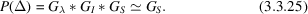For the cases considered here, the focus will be on sample-broadening models that allow improved fits within the context of a Rietveld refinement; a more detailed treatment aimed at extracting sample characteristics (e.g. crystallite size distributions) is covered in Chapter 5.1.

Two mechanisms for sample broadening are considered here: crystallite size and `microstrain' broadening; each will be discussed in turn.

#### 3.3.5.1. Crystallite size broadening

| top | pdf |

The reciprocal space associated with an ideal large crystal will consist of a periodic array of infinitely sharp δ functions, one for each of the structure factors, as expected from the Fourier transform of the essentially infinite and periodic crystal lattice. For real crystals, this limit is reached for crystal dimensions exceeding circa 10 µm. The Fourier transform of a crystal lattice that is smaller than this will show a profile that follows the form described by the sinc(x) = sin(πx)/πx function. Any dispersion in the crystal sizes in a powder sample will smear this into a form intermediate between a Gaussian and a Lorentzian, which is well described by either a Voigt [equation (3.3.9)], a pseudo-Voigt [equation (3.3.8)] or the less-useful Pearson VII [equation (3.3.7)] function. The physical process used to form the powder will influence the details of the size distribution; usually this will approximate a log-normal distribution and the resulting peak-shape contribution from crystallite size effects will be largely Lorentzian with a width ΓSL. Predominantly Gaussian size broadening can only occur if the size distribution is very tightly monodisperse. Then, for isotropic crystal dimensions this broadening is uniformly the same everywhere in reciprocal space; e.g. Δd* = constant ≃ 1/p, where p is the crystallite size. Transformation via Bragg's law to the typical measurement of a powder pattern as a function of 2θ gives this Lorentzian width as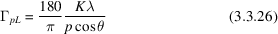expressed in degrees and the Scherrer constant, K, which depends on the shape of the crystallites; e.g. K = 1 for spheres, 0.89 for cubes etc. (see Table 5.1.1in Chapter 5.1). A similar expression for the crystallite size from a neutron TOF experiment is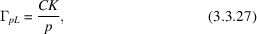where C is defined by equation (3.3.21). In some cases the crystallites have anisotropic shapes (e.g. plates or needles), in which case the peak broadening will be dependent on the respective direction in reciprocal space for each reflection. Many Rietveld refinement programs implement various models for this anisotropy.

#### 3.3.5.2. Microstrain broadening

| top | pdf |

The existence of imperfections (e.g. deformation faults) within the crystal lattice produces local distortions of the lattice and thus a broadening of the points in reciprocal space. To a first approximation these points are broadened proportionally to their distance from the origin, e.g. Δd*/d* = Δd/d ≃ constant.

As for crystallite size, there is normally dispersion in the density of defects and thus the peak shape will be intermediate between a Gaussian and a Lorentzian form, and it is well described by the Voigt, pseudo-Voigt or Pearson VII functions. Usually, the Lorentzian form dominates this type of broadening and it is the most common form of sample broadening in powder diffraction. It usually arises because of defects introduced during sample preparation (especially during grinding). The Lorentzian width contribution from microstrain broadening is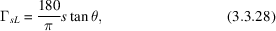where s is the dimensionless microstrain; it is frequently multiplied by 106. A similar expression for neutron TOF is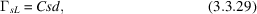where C is defined by equation (3.3.21).

In many cases, the microstrain broadening is not isotropic; presumably this is a consequence of the interaction between the defects and the elastic properties of the crystals. A phenomenological description of these effects by Popa (1998) and Stephens (1999) is obtained by considering the variance of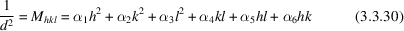with respect to each of the coefficients αi.where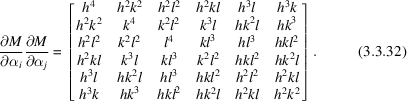Examination of this sum for the triclinic case collects terms to give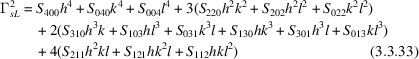with 15 coefficients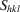. The subscript hkl in Shkl refers to the powers used forin equations (3.3.33)–(3.3.44).

For Laue symmetries other than triclinic, there are restrictions on the allowedterms and, as a practical matter, additional equivalences from symmetry-forced reflection overlaps for trigonal and tetragonal Laue symmetries.

Monoclinic (2/m, b axis unique; others similar, nine coefficients):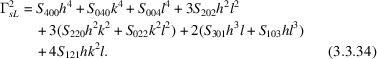Orthorhombic (mmm, six coefficients):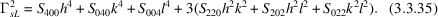Tetragonal (4/m, five coefficients):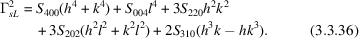The last coefficient (S310) cannot normally be determined owing to exact reflection overlaps. Thus, equation (3.3.37)is normally used for both 4/m and 4/mmm Laue symmetries:

Tetragonal (4/mmm, four coefficients):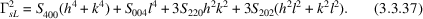Trigonal (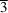, rhombohedral setting, five coefficients):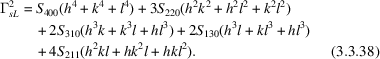The pair of coefficients S310 and S130 cannot normally be independently determined owing to exact reflection overlaps. Thus, equation (3.3.39)is normally used for both rhombohedral symmetries:

Trigonal (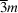, rhombohedral setting, four coefficients):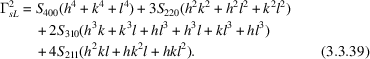Trigonal (, five coefficients):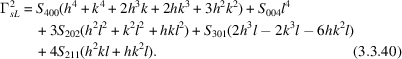The coefficient S301 cannot normally be independently determined owing to exact reflection overlaps. Thus, equation (3.3.42)is normally used forLaue symmetry.

Trigonal (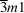, four coefficients):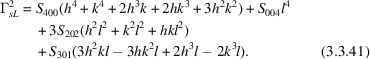The coefficient S301 cannot normally be independently determined due to exact reflection overlaps. Thus, equation (3.3.43)is normally used forLaue symmetry.

Trigonal (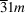, four coefficients):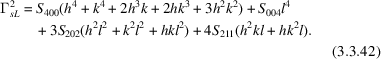Hexagonal (6/m and 6/mmm, three coefficients):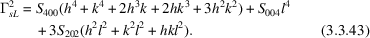Cubic (and, two coefficients):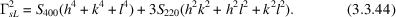These equations can be used with the refined values of the coefficients to produce a surface representing the extent of the microstrain in reciprocal space. The surface resulting from Stephens' (1999) analysis of powder diffraction data from sodium parahydroxybenzoate is shown Fig. 3.3.4. At the present time, the connection between the elastic properties and defects with these microstrain surface models is unclear. Some aspects of this for cubic and hexagonal systems are discussed in Chapter 5.1.Figure 3.3.4 | top | pdf |Microstrain surface for sodium parahydroxybenzoate multiplied by 106.

### References

Finger, L. W., Cox, D. E. & Jephcoat, A. P. (1994). A correction for powder diffraction peak asymmetry due to axial divergence. J. Appl. Cryst. 27, 892–900.Google Scholar
Popa, N. C. (1998). The (hkl) dependence of diffraction-line broadening caused by strain and size for all Laue groups in Rietveld refinement. J. Appl. Cryst. 31, 176–180.Google Scholar
Stephens, P. W. (1999). Phenomenological model of anisotropic peak broadening in powder diffraction. J. Appl. Cryst. 32, 281–289.Google Scholar
Von Dreele, R. B., Jorgensen, J. D. & Windsor, C. G. (1982). Rietveld refinement with spallation neutron powder diffraction data. J. Appl. Cryst. 15, 581–589.Google Scholar